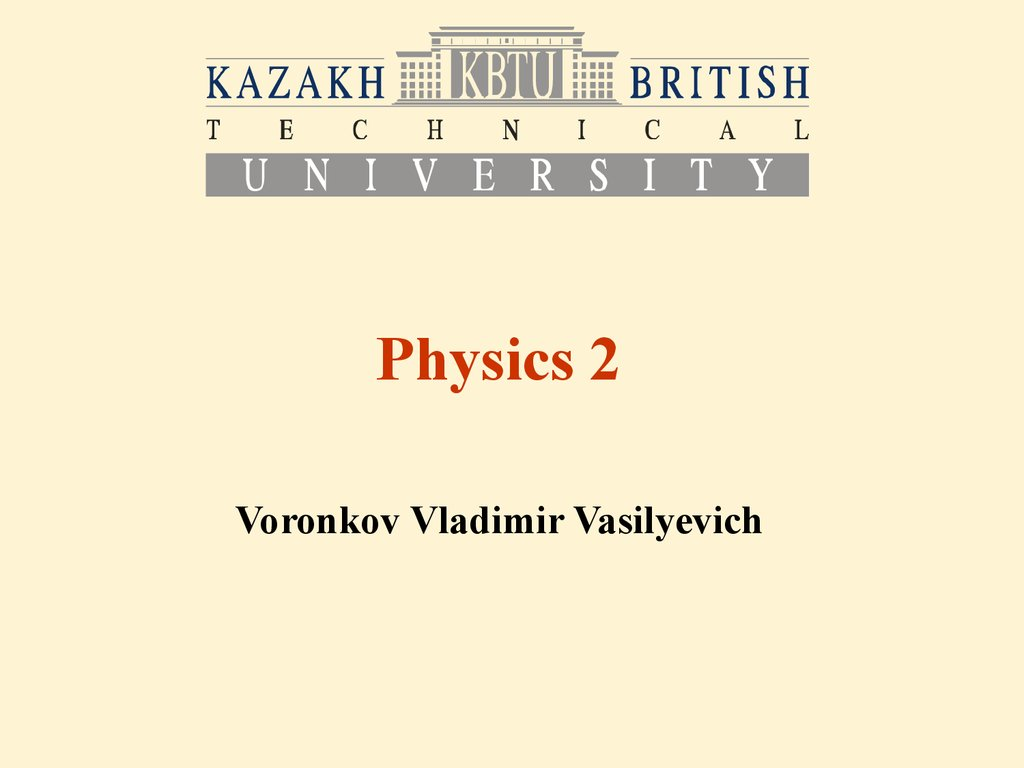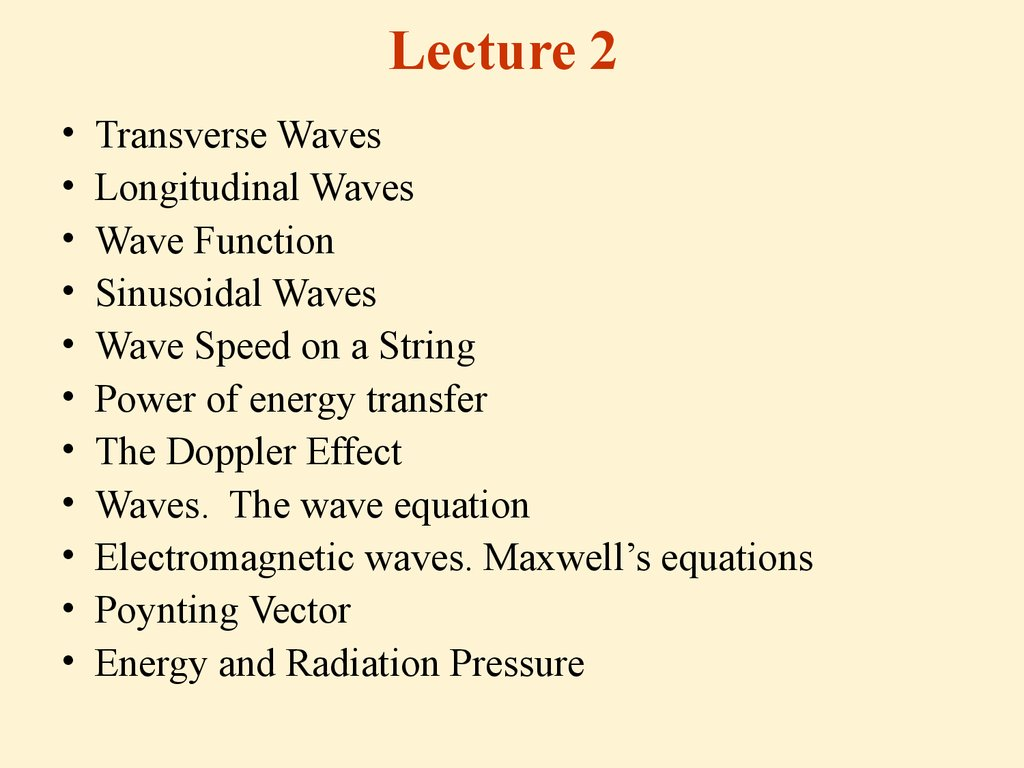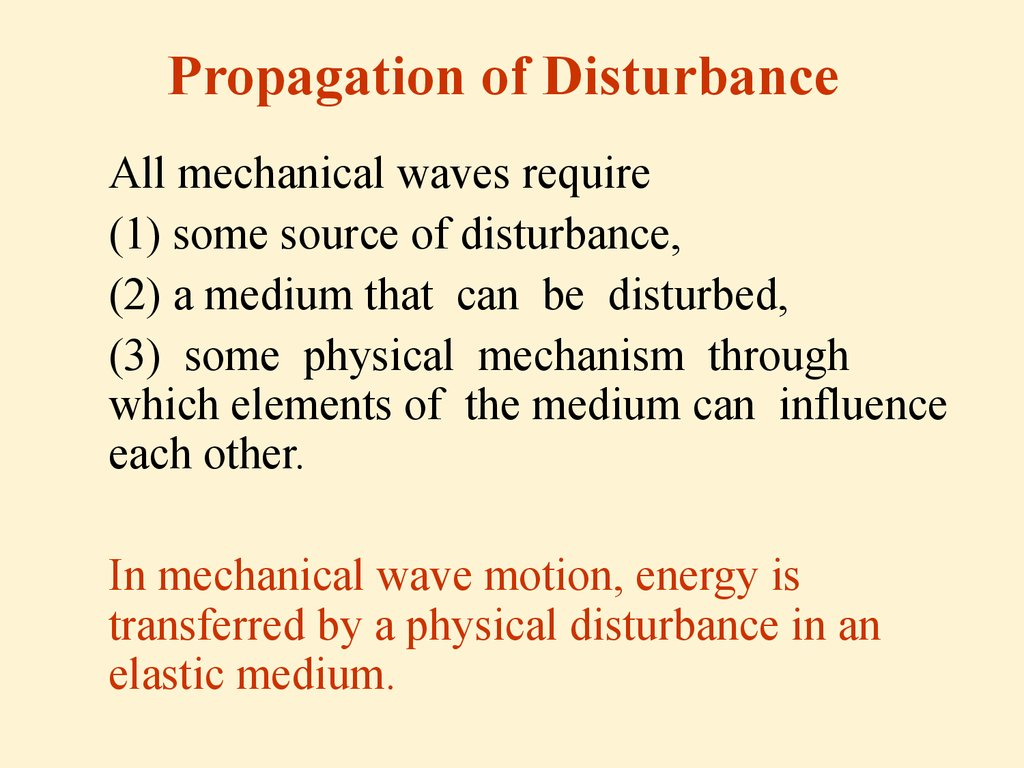# Transverse waves. Longitudinal waves. Energy and radiation pressure

Physics 2

## 2. Lecture 2

Transverse Waves
Longitudinal Waves
Wave Function
Sinusoidal Waves
Wave Speed on a String
Power of energy transfer
The Doppler Effect
Waves. The wave equation
Electromagnetic waves. Maxwell’s equations
Poynting Vector

## 3. Propagation of Disturbance

All mechanical waves require
(1) some source of disturbance,
(2) a medium that can be disturbed,
(3) some physical mechanism through
which elements of the medium can influence
each other.
In mechanical wave motion, energy is
transferred by a physical disturbance in an
elastic medium.

## 4. Transverse waves

A traveling wave or pulse
that causes the elements
of the disturbed medium
to move perpendicular to
the direction of
propagation is called a
transverse wave.

## 5. Longitudinal Waves

• A traveling wave or pulse that causes the
elements of the medium to move parallel to
the direction of propagation is called a
longitudinal wave.

## 6. What Do Waves Transport?

• The disturbance travels or propagates with
a definite speed through the medium. This
speed is called the speed of propagation,
or simply the wave speed.
• Mechanical waves transport energy, but
not matter.

## 7. Wave Function

A one-dimensional pulse traveling to the right with a speed v:
At t = 0, the shape of the
pulse is given by y = f (x).
At some later time t, the
shape remains unchanged
and the vertical position
of an element of the
medium any point P is
given by y = f (x - vt).

## 8.

The shape of the pulse traveling to the right does not change
with time:
y(x,t)=y(x-vt,0)
We can define transverse, or y-positions of elements in the
pulse traveling to the right using f(x) :
y(x,t)=f(x-vt)
And for a pulse traveling to the left:
y(x,t)=f(x+vt)
The function y(x,t) is called the wave function, v is the speed of
wave propagation.
The wave function y(x,t) represents:
- in the case of transverse waves: the transverse position of any
element located at position x at any time t
- in the case of longitudinal waves: the longitudinal
displacement of a particle from the equilibrium position

## 9. Sinusoidal Waves

When the wave function is
sinusoidal then we have
sinusoidal wave.
A one-dimensional sinusoidal wave traveling
to the right with a speed V. The brown curve
represents a snapshot of the wave at t = 0,
and the blue curve represents a snapshot at
some later time t.

## 10.

(a) The wavelength of a wave
is the distance between any
two identical points on
crests or troughs).
The maximum displacement
from equilibrium of an
element of the medium is
called the amplitude A of the
wave.
(b) The period T of a wave is the
time interval required for the
wave to travel one
wavelength.

## 11.

The frequency of a periodic wave is the number of crests (or
troughs, or any other point on the wave) that pass a given
point in a unit time interval:
The sinusoidal wave function at t=0:
The sinusoidal wave function at any t:
If the wave travels to the left then x-vt must be replaced by
x+vt.

## 12.

Then the wave function takes the form:
Let’s introduce new parameters:
Wave number:
Angular frequency:

## 13.

So the wave function is:
Connection of wave speed with other parameters:
The foregoing wave function assumes that the vertical position y
of an element of the medium is zero at x=0 and t=0. This need not
be the case. If it is not, we the wave function is expressed in the
form:
is the phase constant.

## 14. Wave Speed on String

• If a string under tension is pulled sideways and
then released, the tension is responsible for
accelerating a particular element of the string back
toward its equilibrium position. The acceleration of
the element in y-direction increases with increasing
tension, and the wave speed is greater. Thus, the
wave speed increases with increasing tension.
• Likewise, the wave speed should decrease as the
mass per unit length of the string increases.
This is because it is more dif cult to accelerate
a massive element of the string than a light
element.

## 15.

• T is the tension in the string
• is mass per unit length of the string
• Then the wave speed on the string is
• Do not confuse the T in this equation for
the tension with the symbol T used for
the period of a wave.

## 16. Rate of Energy Transfer by Sinusoidal Waves on Strings

Waves transport energy when they propagate through a
medium.
P is the power or rate of energy transfer
m is mass per unit length of the string
is the wave angular frequency
A is the wave amplitude
V is the wave speed
In general, the rate of energy transfer in any
sinusoidal wave is proportional to the square of the
angular frequency and to the square of the
amplitude.

## 17. The Doppler Effect

• Doppler effect is the shift in frequency and wavelength of waves
that results from a relative motion of the source, observer and
medium.
• If the source of sound moves relative to the observer, then the
frequency of the heard sound differs to the frequency of the source:
• f is the frequency of the source
• V is the speed of sound in the media
• VS is the speed of the source relative to the media, positive direction is
toward the observer
• VO is the speed of the observer, relative to the media, positive
direction is toward the source
• f`’ is the frequency heard by the observer
• The Doppler Effect is common for all types of waves: mechanical,
sound, electromagnetic waves.

## 18.

• When the source is stationary with respect
to the medium the wavelength does not
change.
`
• When the source moves with respect to
the medium the wavelength changes:
` -vs/f
• So when the observer is stationary with
respect to the medium and the source
approaches the observer the wavelength
decreases and vice versa.

## 19. Wave Equation

• From the wave function we can get an expression for the transverse
velocity y/ t of any particle in a transverse wave:
y/ t means partial derivative of function y(x,t) by t, keeping x constant.
2 y/ x2 is the second partial derivative of y with respect to x at t
constant.
• y is:
– the transverse displacement of a media particle in the case of transverse waves
– the longitudinal displacement of a media particle from the equilibrium position in
the case of longitudinal waves (or variations in either the pressure or the
density of the gas through which the sound waves are propagating)
– In the case of electromagnetic waves, y corresponds to electric or magnetic field
components.
• x is the displacement of the traveling wave
• V is the wave speed: V=dx/dt

## 20. Electromagnetic Waves

The properties of electromagnetic waves can be
deduced from Maxwell’s equations:
(1)
(2)
(3)
(4)

## 21.

• Equation (1):
• Here integration goes across an enclosed
surface, q is the charge inside it.
• This is the Gauss’s law: the total electric
ux through any closed surface equals the
net charge inside that surface divided by
0.
• This law relates an electric eld to the
charge distribution that creates it.

## 22.

• Equation (2):
• Here integration goes across an enclosed surface.
It can be considered as Gauss’s law in magnetism,
states that the net magnetic ux through a closed
surface is zero.
• That is, the number of magnetic eld lines that enter
a closed volume must equal the number that leave
that volume. This implies that magnetic eld lines
cannot begin or end at any point. It means that there is
no isolated magnetic monopoles exist in nature.

## 23.

• Equation (3):
• Here integration goes along an enclosed path,
ФB is a magnetic flux through that enclosed path.
• This equation is Faraday’s law of induction,
which describes the creation of an electric
eld by a changing magnetic ux.
• This law states that the emf, which is the line
integral of the electric eld around any
closed path, equals the rate of change of
magnetic ux through any surface area
bounded by that path.

## 24.

• Equation (4):
• This is Ampère–Maxwell law, or the generalized
form of Ampère’s law. It describes the creation
of a magnetic eld by an electric eld and
electric currents. the line integral of the
magnetic eld around any closed path is the
sum of 0 times the net current through that
path and 0 0 times the rate of change of
electric ux through any surface bounded by
that path.

## 25. Plane-Wave Assumption

We assume that an electromagnetic wave travels
in the x-direction. In this wave, the electric eld
E is in the y-direction, and the magnetic eld B is
in the z-direction. Waves such as this one, in which
the electric and magnetic elds are restricted to
being parallel to a pair of perpendicular axes, are
said to be linearly polarized waves. Furthermore, we
assume that at any point in space, the magnitudes E
and B of the elds depend upon x and t only, and not
upon the y or z coordinate.

## 26.

An electromagnetic wave traveling at velocity
c in the positive x-direction. The electric eld
is along the y-direction, and the magnetic eld
is along the z-direction. These elds depend
only on x and t.

## 27.

• In empty space there is no currents and free charges:
I=0, q=0, then the 4-th Maxwell’s equation turns
into:
• Using it with the 3-d Maxwell’s equation
and the plane-wave assumption, we obtain the
following differential equations relating E and B:

## 28.

And eventually we obtain:
These two equations both have the form of the
general wave equation with the wave speed v
replaced by c, the speed of light:

## 29.

• 0 is the free space magnetic permeability:
• 0 is the free space electric permeability:
• c is the speed of light in vacuum:

## 30.

So we obtained the wave equations for electromagnetic
waves:
The simplest solution to those is a sinusoidal wave:
Using this solution, we can derive that
That is, at every instant the ratio of the magnitude of
the electric eld to the magnitude of the magnetic eld
in an electromagnetic wave equals the speed of light.

## 31. Electromagnetic Waves Properties (Summary)

• The solutions of Maxwell’s third and fourth equations
are wave-like, with both E and B satisfying a wave
equation.
• Electromagnetic waves travel through empty space at the
speed of light c.
• The components of the electric and magnetic elds of plane
electromagnetic waves are perpendicular to each other and
perpendicular to the direction of wave propagation. So,
electromagnetic waves are transverse waves.
• The magnitudes of E and B in empty space are related
by the expression E/B = c.
• Electromagnetic waves obey the principle of superposition.

## 32. Poynting Vector

The rate of flow of energy in electromagnetic waves is
• S is called the Poynting vector. The magnitude of
the Poynting vector represents the rate at which
energy ows through a unit surface area perpendicular
to the direction of wave propagation. Thus, the
magnitude of the Poynting vector represents power
per unit area. The direction of the vector is along the
direction of wave propagation.

## 33. Energy of Electromagnetic Waves

Electromagnetic waves carry energy with total
instantaneous energy density:
This instantaneous energy is carried in equal
amounts by the electric and magnetic fields:

## 34.

When this total instantaneous energy density is
averaged over one or more cycles of an
electromagnetic wave, we obtain a factor of 1/2.
Hence, for any electromagnetic wave, the total
average energy per unit volume is

## 35. Pressure of Electromagnetic Waves

Electromagnetic waves exert pressure on the
surface. If the surface is absolutely absorbing, then
the pressure per unit area of the surface is
In the case of absolutely reflecting surface, the
pressure per unit area of the surface doubles:

## 36. Units in Si

• Wavenumber
• Phase constant
• Poynting vector
k
S